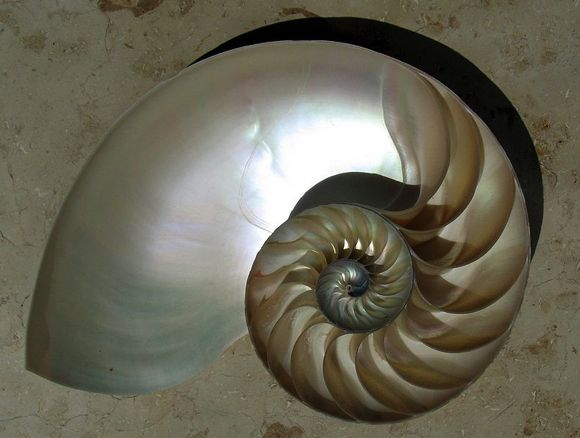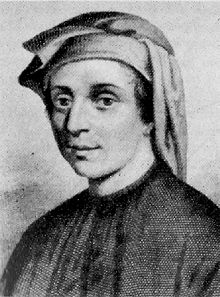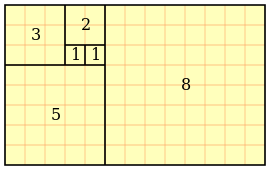## What’s special about the shape of a Nautilus shell?

The early mathematician Fibonacci introduced Arabic numerals to the West. He also discovered a number sequence that’s in everything from daisies to databases.

EarthSky community member Norman Straight asked:

Who is Fibonacci, and how does his work relate to the shape of a Nautilus shell?Cutaway of a Nautilus shell showing the chambers arranged in an approximately logarithmic spiral. Image via Wikipedia’s Mathematics Portal.Fibonacci via Wikimedia Commons

Leonardo Pisano Bigollo (c. 1170 – c. 1250) – aka Leonardo of Pisa or sometimes just Fibonacci – was one of the most famous mathematicians in the Middle Ages. He initiated the spread of the Hindu–Arabic numeral system to Europe, in his book Liber Abaci (Book of Calculation), published in the year 1202. This number system is based on 10 different glyphs or symbols, including a zero. It’s the system we use every day: 1, 2, 3, 4, 5, 6, 7, 8, 9, 0.

Fibonacci also laid the groundwork for our modern-day mathematical understanding of certain shapes in nature, including Nautilus shells. In his book, Fibonacci introduced what’s now called the Fibonacci number or sequence, which can be described as follows.

Suppose you place two baby rabbits in a garden. The pair, at one month old, is too young to reproduce. Suppose that in their second month, and every month after that, they produce two more rabbits. If each new pair of rabbits does the same, the number of pairs each month increases according to the following sequence, starting with 0 and 1. This sequence begins 0, 1, 1, 2, 3, 5, 8, 13, 21, 34, 55, 89, 144, 233, 377, 610, 987. Each number is the sum of the two previous numbers.An approximation of a logarithmic spiral, created by drawing circular arcs connecting the opposite corners of squares in the Fibonacci tiling; this one uses squares of sizes 1, 1, 2, 3, 5, 8, 13, 21, and 34. Image via Wikipedia.A tiling with squares whose side lengths are successive Fibonacci numbers via Wikipedia

Mathematicians have learned to use Fibonacci’s sequence to describe certain shapes that appear in nature. These shapes are called logarithmic spirals, and Nautilus shells are just one example. You also see logarithmic spiral shapes in spiral galaxies, and in many plants such as sunflowers.

Nature forms these spirals in the most efficient way possible, and mathematicians have learned to describe them, using Fibonacci’s sequence.

Bottom line: Leonardo Pisano Bigollo, aka Leonardo of Pisa or sometimes just Fibonacci, is best known in the modern world for spreading the Hindu–Arabic numeral system in Europe. He also introduced the west to what is now called Fibonacci’s number or sequence, which can be used to describe certain shapes found in nature: spiral galaxies, sunflowers, Nautilus shells.

See a movie that demonstrates how to construct a Fibonacci spiral.

1999 article mathematician Keith Devlin: New mathematical constant discovered

Fibonacci Flimflam via Lock Haven University

Who was Fibonacci? via University of Surrey

Scientists look to sunflowers for solar panel design

### Deborah Byrd